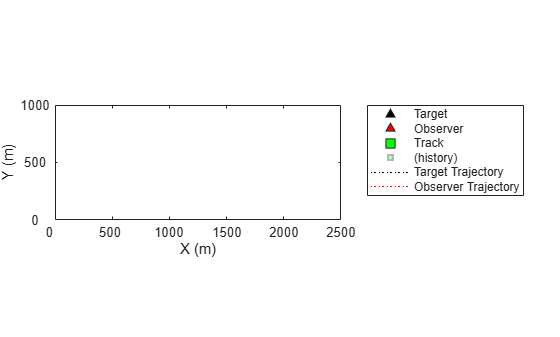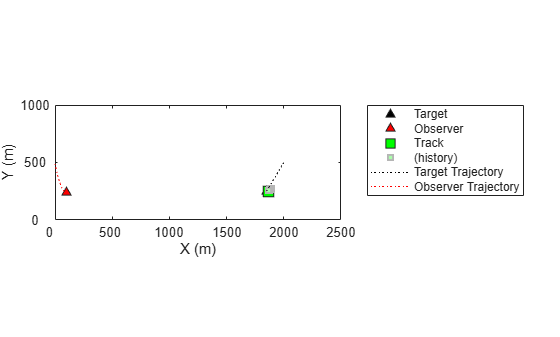Documentation

initcvmscekf

Constant velocity trackingMSCEKF initialization

Description

example

mscekf = initcvmscekf(detection) initializes a trackingMSCEKF class (extended Kalman filter for tracking in modified spherical coordinates) based on information provided in an objectDetection object, detection. The function assumes a target range of 3e4 units and a range-covariance of 1e10 units2.

The trackingMSCEKF object can be used with trackers for tracking targets with angle-only measurements from a single observer.

example

mscekf = initcvmscekf(detection,rangeEstimation) allows specifying the range information to the filter. The rangeEstimation variable is a two-element vector, where the first element specifies the range of the target, and the second element specifies the standard deviation in range.

Examples

collapse all

Create an angle-only detection.

detection = objectDetection(0,[30;20],'MeasurementParameters',...
struct('Frame','Spherical','HasRange',false));

Use initcvmscekf to create a trackingMSCEKF filter initialized using the angle-only detection.

filter = initcvmscekf(detection)
filter =
trackingMSCEKF with properties:

State: [6×1 double]
StateCovariance: [6×6 double]

StateTransitionFcn: @constvelmsc
StateTransitionJacobianFcn: @constvelmscjac
ProcessNoise: [3×3 double]
ObserverInput: [3×1 double]

MeasurementFcn: @cvmeasmsc
MeasurementJacobianFcn: @cvmeasmscjac
MeasurementNoise: [2×2 double]

Create measurement parameters for subsequent rotation.

measParamSensorToPlat = struct('Frame','Spherical','HasRange',false,...
'Orientation',rotmat(quaternion([0 0 30],'rotvecd'),'frame'))
measParamSensorToPlat = struct with fields:
Frame: 'Spherical'
HasRange: 0
Orientation: [3×3 double]

measParamPlatToScenario = struct('Frame','Rectangular','HasRange',false,...
'Orientation',rotmat(quaternion([30 0 0],'rotvecd'),'frame'))
measParamPlatToScenario = struct with fields:
Frame: 'Rectangular'
HasRange: 0
Orientation: [3×3 double]

measParam = [measParamSensorToPlat;measParamPlatToScenario];
detection = objectDetection(0,[30;20],'MeasurementParameters',measParam);

Initialize a filter.

filter = initcvmscekf(detection);

Check that filter's measurement is same as detection.

cvmeasmsc(filter.State,measParam)
ans = 2×1

30.0000
20.0000

Consider a scenario when the target is moving at a constant velocity along and the observer is moving at a constant acceleration. Define target's initial state using a constant velocity model.

tgtState = [2000;-3;500;-5;0;0];

Define observer's initial state using a constant acceleration model.

observerState = [0;2;0;490;-10;0.2;0;0;0];

Create a trackerGNN object to use with initcvmscekf with some prior information about range and range-covariance.

range = 1000;
rangeStdDev = 1e3;
rangeEstimate = [range rangeStdDev];
tracker = trackerGNN('FilterInitializationFcn',@(det)initcvmscekf(det,rangeEstimate));

Simulate synthetic data by using measurement models. Get az and el information using the cvmeas function.

syntheticParams = struct('Frame','Spherical','HasRange',false,...
'OriginPosition',observerState(1:3:end));
meas = cvmeas(tgtState,syntheticParams);

Create an angle-only objectDetection to simulate synthetic detection.

detection = objectDetection(0,meas,'MeasurementParameters',...
struct('Frame','Spherical','HasRange',false),'MeasurementNoise',0.033*eye(2));

Create trackPlotter and platformPlotter to visualize the scenario.

tp = theaterPlot('XLimits',[0 2500],'YLimits',[0 1000]);
targetPlotter = platformPlotter(tp,'DisplayName','Target','MarkerFaceColor','k');
observerPlotter = platformPlotter(tp,'DisplayName', 'Observer','MarkerFaceColor','r');
trkPlotter = trackPlotter(tp,'DisplayName','Track','MarkerFaceColor','g','HistoryDepth',50);
tgtTrajPlotter = trajectoryPlotter(tp,'DisplayName','Target Trajectory','Color','k');
obsTrajPlotter = trajectoryPlotter(tp,'DisplayName','Observer Trajectory','Color','r');Run the tracker.

time = 0; dT = 0.1;
tgtPoses = [];
obsPoses = [];
while time < 50
[confTracks,tentTracks,allTracks] = tracker(detection,time);
for i = 1:numel(allTracks)
setTrackFilterProperties(tracker,allTracks(i).TrackID,'ObserverInput',observerState(3:3:end));
end

% Update synthetic detection.
observerState = constacc(observerState,dT);
tgtState = constvel(tgtState,dT);
syntheticParams.OriginPosition = observerState(1:3:end);
detection.Measurement = cvmeas(tgtState,syntheticParams);
time = time + dT;
detection.Time = time;

% Update plots
tgtPoses = [tgtPoses;tgtState(1:2:end)']; %#ok
obsPoses = [obsPoses;observerState(1:3:end)']; %#ok
targetPlotter.plotPlatform(tgtState(1:2:end)');
observerPlotter.plotPlatform(observerState(1:3:end)');
tgtTrajPlotter.plotTrajectory({tgtPoses});
obsTrajPlotter.plotTrajectory({obsPoses});
% Plot the first track as there are no false alarms, this should be
% the target.
% Get positions from the MSC state of the track.
cartState = cvmeasmsc(allTracks(i).State,'rectangular') + observerState(1:3:end);
trkPlotter.plotTrack(cartState');
endInput Arguments

collapse all

Detection report, specified as an Object Detections object.

Example: detection = objectDetection(0,[1;4.5;3],'MeasurementNoise', [1.0 0 0; 0 2.0 0; 0 0 1.5])

Range information, specified as a two-element vector, where the first element specifies the range of the target, and the second element specifies the standard deviation in range.

Data Types: single | double

Output Arguments

collapse all

Constant velocity tracking extended Kalman filter in an MSC frame, returned as a trackingMSCEKF object.

Algorithms

• The function configures the filter with process noise assuming a unit target acceleration standard deviation.

• The function configures the covariance of the state in an MSC frame by using a linear transformation of covariance in a Cartesian frame.

• You can use this function as the FilterInitializationFcn property of trackerTOMHT and trackerGNN System objects.

• The function initializes the ObserverInput of the trackingMSCEKF class with zero observer acceleration in all directions. You must use the setTrackFilterProperties function of the trackers to update the ObserverInput.

Extended Capabilities

C/C++ Code GenerationGenerate C and C++ code using MATLAB® Coder™.